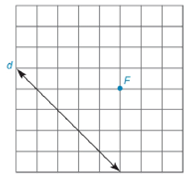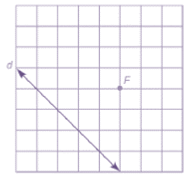Chapter 7.1, Problem 27EElementary Geometry For College St...

7th Edition
Alexander + 2 others
ISBN: 9781337614085

Solutions

Chapter
SectionElementary Geometry For College St...

7th Edition
Alexander + 2 others
ISBN: 9781337614085
Textbook Problem

Note: Exercises preceded by an asterisk are of a more challenging nature.For Exercises 23 to 28, use the grid and your compass (as needed) to locate several points on the parabola having the given focus F and directrix d . Then sketch the parabola that is characterized by these points.To determine

To sketch:

The parabola using the below given grid with directrix and focus.Explanation

Definition:

A parabola is the locus of points that are equidistant from a fixed line and a fixed point not on that line.

The fixed point is known as focus of the parabola and the fixed line is known as directrix of the parabola.

Calculation:

Procedure:

1. Draw the given directrix d and focus F and locate a point D1.

2. Locate a point P1 which is the mid-point of FD1. The point P1 will be the vertex.

3. Locate the points P1, P2, and P3 such that D

Still sussing out bartleby?

Check out a sample textbook solution.

See a sample solution

The Solution to Your Study Problems

Bartleby provides explanations to thousands of textbook problems written by our experts, many with advanced degrees!

Get Started

In Exercise 11-14, factor the expression. x2+5x+6

Calculus: An Applied Approach (MindTap Course List)

In Exercises 63-68, use the graph of the function f to determine limxf(x) and limxf(x) 64.

Applied Calculus for the Managerial, Life, and Social Sciences: A Brief Approach

Show that quadrilateral ABCD is a parallelogram.

Elementary Geometry for College Students

True or False: x2 + 6x + 2y = 1 defines y as a function of x.

Study Guide for Stewart's Single Variable Calculus: Early Transcendentals, 8th